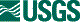## ISIS 2ISIS Application Documentation

# qview

Printer Friendly View | TOC | Home

Display and analyze cubes

 Overview Parameters

## Description

This program will display cubes and allow for interactive analysis. Although the program comprises a number of individual tools to perform analysis on your data, only the Spatial Plot Tool is discussed here until further notice.

### Spatial Plot Tool

This tool is used for analyzing manually selected pixel DN values. It works with two different modes: linear and rotated rectangle. It is also capable of handling three different modes of interpolation: Nearest Neighbor, BiLinear and Cubic Convolution.

#### Linear

This mode involves drawing a line across an open cube. The selection is started by clicking and holding the mouse where you would like to start your calculations, at which point you may drag the mouse in any direction and release the click to establish the end of your selection.

The tool calculates the length of the line in pixels, rounding to the nearest full pixel value. It then divides the actual length of the line by this rounded length to achieve a step-size. Please note: This step size may be slightly bigger or smaller than a pixel length, but this difference becomes negligible for larger selections. For example, if the length of the drawn line was 2.91 pixels, the tool would round the length to 3 and the step-size would be 2.91/3 (or 0.97).

A plot window will be generated with the calculated DN values of the pixels. The first value to be calculated is the value where the original mouse click was made. At each increment, according to step size, the tool calculates the value along the line until it reaches where the mouse-click was released. This means that for a line with a rounded length of 3, the plot would show a total of 4 plotted DN values. Please note: For a line with a rounded length of n, the tool will calculate n + 1 values.

#### Rotated Rectangle

This mode of the Spatial Plot Tool involves drawing a rectangle across an open cube. The selection starts by clicking and holding the mouse where you would like to start your calculations, at which point you may drag the mouse in any direction and release the click to establish one edge of your selection. You may then drag the mouse away from this original line to expand in the other direction. A single mouse click establishes your final selection. Please note: The tool will lock angles to be perpendicular and so your selections will always be a perfect rectangle.

Similar to the linear mode of this tool, the lengths of the lines in pixels are rounded to the nearest full pixel value, and then the lengths are divided by their rounded lengths to establish a step-size. Please note: The step-size along one edge of the selection may differ from the step-size of the perpendicular edge, this becomes negligible for larger selections. For example, if the length of the line in one direction was 2.91 pixels, the step-size would be 2.91/3 (or 0.97), whereas if the length of the line in the perpendicular direction was 4.92, the step size would be 4.92/5 (0.98).

A plot window will be generated with the calculated DN values. This is done by calculating the value where the original mouse click was made, then continuing in the direction of that first-drawn line, calculating the DN value at each step-sized increment until it reaches where the original mouse-click was released. The tool will then calculate the average of these values. This average becomes the first value in the plot.

The tool will then shift a step-size along the perpendicular edge, and calculate the average DN value along a line running parallel to the first-drawn line. This average becomes the second value in the plot. The tool continues this pattern until it has reached the opposite edge of the rectangle as the first-drawn line.

#### Interpolations

Spatial Plot Tool makes use of Nearest Nieghbor, BiLinear and Cubic Convolution interpolations when calculating the DN values at particular points. Do be aware that when the tool is calculating a DN value at a particular point, it is calculating the interpolated DN value at that point.